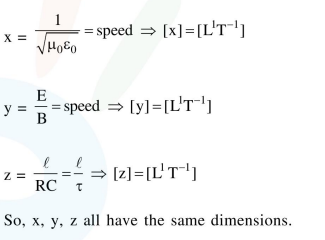# The quantities

Question:

The quantities $\quad x=\frac{1}{\sqrt{\mu_{0} \in_{0}}}, y=\frac{E}{B} \quad$ and $\mathrm{z}=\frac{1}{\mathrm{CR}}$ are defined where $\mathrm{C}$-capacitance,

R-Resistance, $l$-length, E-Electric field, $B$-magnetic field and $\in_{0}, \mu_{0},-$ free space permittivity and permeability respectively. Then:

1. Only $x$ and $y$ have the same dimension

2. $x, y$ and $z$ have the same dimension

3. Only $x$ and $z$ have the same dimension

4. Only y and z have the same dimension

Correct Option: , 2

Solution: